Tuesday,  April 27th

Last class we looked at the cone as related to projection from a central light source and the curves determined by conic sections.
Finish watch video on conics.
The characterization of points on a conic by measurements are:
ellipse:  AC + BC = constant
parabola: EC = EF
hyperbola: |CB-CA| = constant.
Rope Conic Activity.
Using a rope form a human ellipse.
After that  demonstrate how to use a rope to form a hyperbola and then a parabola.

String drawing conic activity.

ellipse:  AC + BC = constant
parabola: EC = EF
hyperbola: |CB-CA| = constant.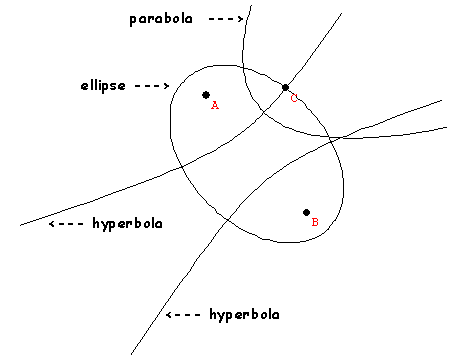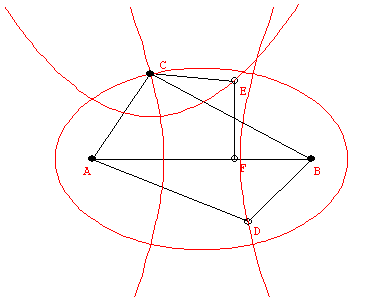The Conics Video shows how the conics are connected to these measurement qualities.

Key background: Tangents to a circle are equal in length.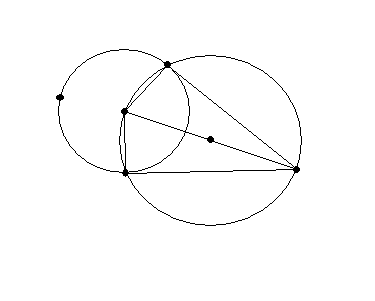In  right triangle, the ratios of  the length of the side
opposite an angle to the hypotenuse length depends only on the angle.
This ratio is called the sine of the angle and is abbreviated "sin".

If the angle is designated by the vertex A in a right triangle ABC where the right angle is at vertex C, then sin(A) = BC/AB.

Examples:
If A is a 45 degree angle, sin(A) = 1/ sqrt(2).
If A is a 30 degree angle, sin(A) = 1/2.

Show "Conics" video.

Projective geometry: The study of properties of figures that are related by projections... perspectivities and projectivities.

Examples: The projection of a line is a line. The point of intersection of two lines will project to the point of intersection of the projected lines.

Desargues' Theorem in Space:

We define a perspective relation:
Two points P and P' are perspectively related by the center O if O is on the line PP" . Two triangles ABC and A'B'C' are perspectively related by the center O if O is on the lines AA', BB', and CC'.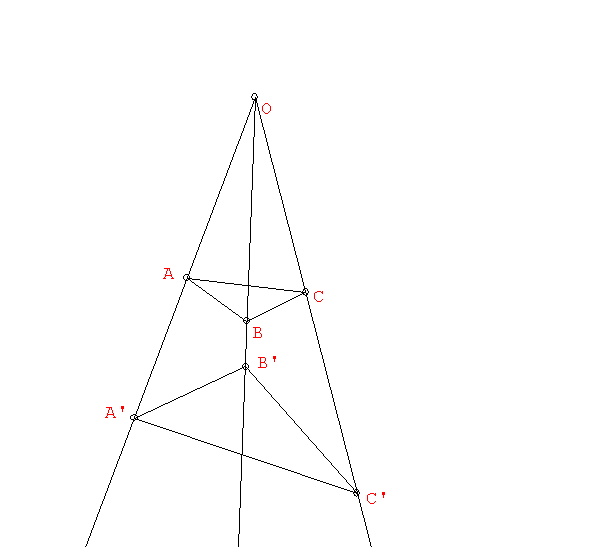Another aspect of Projection: Desargues' Theorem in 3-space and the plane.

Maps of the earth that use Projection:What is projection for Flatland?

Center of projection/ perspective.
[ compare with similarity].
Correspondences:  Activity:Circle to Line:

How to see the Infinite using Projective geometry.

Circle to line and line to Circle!
Line to Line
Perspective and projective relations between points on lines.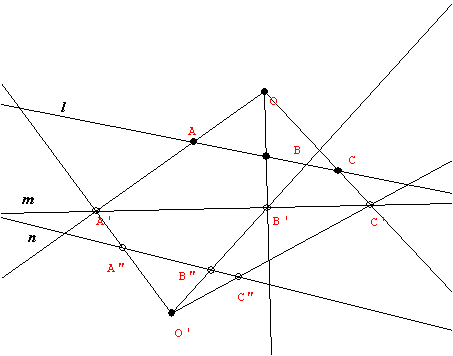Activity on Perspective and Projective relations between points on lines.

Perpective between planes in Space!
Seeing the horizon on a plane.... a line of ideal/infinite points.
How parallel lines "meet" on the horizon.
Equations for conics in coordinate geometry.

Using coordinates to draw in perspective.
Activity: Creating coordinates in the projective plane and using them to transfer a circle to the projective plane as an ellipse.

What do the conics look like in a perspective drawing or in the projective plane?Equations for conics in coordinate geometry.
Using coordinates to draw in perspective.

What do the conic look like in a perspective drawing or in the projective plane?